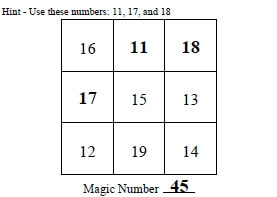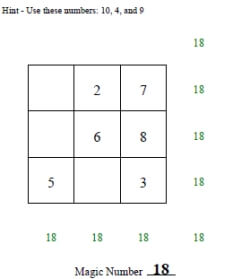Worksheets and No Prep Teaching Resources
Math Worksheets

# Magic Squares Worksheets

Students need a little bit of magic in math, so why not introduce them to these fantastic magic squares worksheets? These creative worksheets will give students excellent practice in the four basic operations and logic. What an exciting, new way for them to practice their skills! They will be having so much fun that they won't even realize they are improving their math square by square!3 by 3 Magic Squares

Magic Number Given - Numbers 1 to 9
Magic Number Not Given - Mix of Different Positive Numbers
Magic Number Not Given - Negative Numbers

3 by 3 Magic Squares and Super Silly Squares Mix

3 by 3 Magic Squares and Super Silly Squares - Numbers 1 to 9
3 by 3 Magic Squares and Super Silly Squares - Mix of Different Positive NumbersPositive numbers: 2 blanks (first answer given; hint for numbers to use given) Positive numbers: 2-3 blanks (first answer given; hint for numbers to use given) Positive numbers: 2-3 blanks (first answer given; hint for numbers to use given; 3 pages for each key) Positive numbers: 3-4 blanks (first answer given; hint for numbers to use given)

4 by 4 Magic Squares

Magic Number Given - Numbers 1 to 9

Super Silly Squares

Subtraction Squares

Multiplication Squares

Mixed Math Square Puzzles

Have a suggestion or would like to leave feedback?